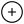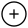# All you need to know about Segment Tree in Programming

22 Aug, 2021

In computer science, a segment tree also known as a statistic tree is a tree data structure used for storing information about intervals, or segments.

It allows querying which of the stored segments contain a given point. It is, in principle, a static structure; that is, it's a structure that cannot be modified once it's built. A similar data structure is the interval tree.

A segment tree is a binary tree. The root of the tree represents the whole array. The two children of the root represent the first and second halves of the array.

Similarly, the children of each node correspond to the two halves of the array corresponding to the node.

We build the tree bottom-up, with the value of each node being the "minimum" (or any other function) of its children's values. This will take `O(n log n)` time.

The number of operations done is the height of the tree, which is `O(log n)`. To do range queries, each node splits the query into two parts, one sub-query for each child.

If a query contains the whole subarray of a node, we can use the precomputed value at the node. Using this optimization, we can prove that only `O(log n)` minimum operations are done.##### Application of Segment Tree
• A segment tree is a data structure designed to perform certain array operations efficiently - especially those involving range queries.
• Applications of the segment tree are in the areas of computational geometry, and geographic information systems.
• The current implementation of Segment Tree implies that you may pass any binary (with two input params) function to it and thus you're able to do range queries for a variety of functions.
Programming Segment Tree Programming competitivecoding Competitive Programming
You need to be Logged in to Comment.# All you need to know about Segment Tree in Programming

22 Aug, 2021

In computer science, a segment tree also known as a statistic tree is a tree data structure used for storing information about intervals, or segments.

It allows querying which of the stored segments contain a given point. It is, in principle, a static structure; that is, it's a structure that cannot be modified once it's built. A similar data structure is the interval tree.

A segment tree is a binary tree. The root of the tree represents the whole array. The two children of the root represent the first and second halves of the array.

Similarly, the children of each node correspond to the two halves of the array corresponding to the node.

We build the tree bottom-up, with the value of each node being the "minimum" (or any other function) of its children's values. This will take `O(n log n)` time.

The number of operations done is the height of the tree, which is `O(log n)`. To do range queries, each node splits the query into two parts, one sub-query for each child.

If a query contains the whole subarray of a node, we can use the precomputed value at the node. Using this optimization, we can prove that only `O(log n)` minimum operations are done.##### Application of Segment Tree
• A segment tree is a data structure designed to perform certain array operations efficiently - especially those involving range queries.
• Applications of the segment tree are in the areas of computational geometry, and geographic information systems.
• The current implementation of Segment Tree implies that you may pass any binary (with two input params) function to it and thus you're able to do range queries for a variety of functions.
Programming Segment Tree Programming competitivecoding Competitive Programming